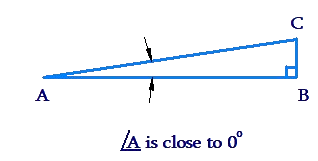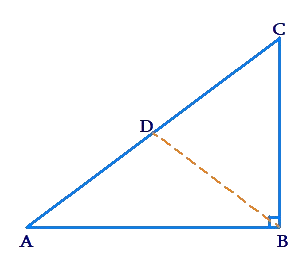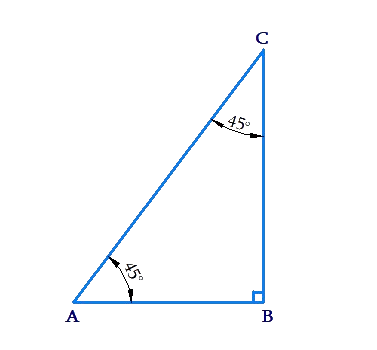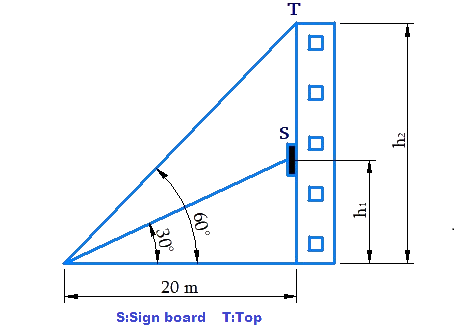# Trigonometric Ratios of Specific Angles

Trigonometric Ratios of Specific Angles

We can determine the trigonometric ratios for the following five angles based on our existing knowledge of pure geometry: $${0^ \circ},\,{30^ \circ },\,{45^\circ },\,{60^\circ }\,\rm{and}\,{90^\circ}$$. Let us see how:

## Trigonometric Ratios of $${0^\circ }\,\rm{and}\,{90^\circ}$$

Consider a $$\Delta ABC$$ which is right-angled at $$B$$, such that $$\angle A$$ is very small:✍Note: As $$\angle A$$ is close to $${0^ \circ, }$$ $$\angle C$$ is close to $${90^ \circ. }$$

Now,

• $$\sin A = \frac{{BC}}{{AC}}$$ will be very small (close to 0), because $$BC$$ is very small.
• $$\cos A = \frac{{AB}}{{AC}}$$ will be almost equal to 1, because the base $$AB$$ is almost equal to the hypotenuse $$AC$$.

Visualize what would happen if $$\angle A$$ was made smaller and smaller until it became $${0^ \circ }$$.

In this scenario,

• $$\sin A$$ would become exactly 0.
• $$\cos A$$  would become exactly 1.

Thus, we conclude that

$\sin {0^\circ } = 0,\,\,\,\,\,\cos {0^\circ } = 1$

If we analyze the sine and cosine of $$\angle C$$ in the same situation, we can conclude that

$\sin {90^ \circ } = 1,\,\,\,\,\,\cos {90^ \circ} = 0$

We summarize these findings below:

$\boxed { \begin{gathered} \sin 0^\circ = 0,{\text{ }}\sin 90^\circ = 1 \hfill \\ \cos 0^\circ = 1,{\text{ }}\cos 90^\circ = 0 \hfill \\ \end{gathered} }$

## Trigonometric Ratios of $${30^ \circ }\,\,\rm{and}\,\,{60^\circ }$$

Consider a $$\Delta ABC$$, which is right-angled at $$B$$. Suppose that $$AC$$ is twice that of $$BC$$:Let $$D$$ be the mid-point of $$AC$$. We can show in plane geometry that $$AD = CD = BD$$, and as a consequence, $$\angle A = {30^ \circ}$$ and $$\angle C = {60^ \circ}$$. You are urged to prove this assertion once again.

⚡Tip: Produce $$BD$$ to a point $$E$$ such that $$BD = DE$$ and then join $$AE$$. After that, prove $$\Delta EDA$$ and $$\Delta BDC$$ congruent.

Now, we have:

\begin{align}\sin A = \sin {30^\circ } &= \frac{{BC}}{{AC}} \hfill \\ &= \frac{{BC}}{{2BC}} \hfill \\ &= \frac{1}{2}\end{align}
_____________________
\begin{align}\cos C = \cos {60^ \circ } = \frac{{BC}}{{AC}} = \frac{1}{2}\end{align}
_____________________
\begin{align}\cos A = \cos{30^\circ } &= \frac{{AB}}{{AC}} \hfill \\ &= \sqrt {1 - {{\left( {\frac{{BC}}{{AC}}} \right)}^2}} \hfill \\ &= \frac{{\sqrt 3 }}{2}\end{align}
_____________________
\begin{align}\sin C = \sin {60^\circ } = \frac{{AB}}{{AC}} = \frac{{\sqrt 3 }}{2}\end{align}
_____________________

To summarize,

$\boxed {\begin{gathered} \sin 30^\circ = \frac{1}{2},{\text{ }}\sin 60^\circ = \frac{{\sqrt 3 }}{2} \hfill \\ \cos 30^\circ = \frac{{\sqrt 3 }}{2},{\text{ }}\cos 60^\circ = \frac{1}{2} \hfill \\ \end{gathered} }$

## Trigonometric Ratios of $${45^\circ }$$

Consider $$\Delta ABC$$ which is right-angled at $$B$$, with $$\angle A = {45^ \circ}.$$ We note that $$\angle C$$ will also be $${45^ \circ}$$ and the triangle will be isosceles:Note that \begin{align}AB = BC = \frac{{AC}}{{\sqrt 2 }}\end{align} (how?), and thus

• \begin{align}\sin {45^\circ } = \,\frac{{BC}}{{AC}} = \frac{1}{{\sqrt 2 }}\end{align}
• \begin{align}\cos {45^ \circ } = \frac{{AB}}{{AC}} = \frac{1}{{\sqrt 2 }}\end{align}

To summarize,

$\boxed {\sin {45^ \circ } = \frac{1}{\sqrt2},\,\,\cos {45^\circ } = \frac{1}{\sqrt2}}$

## Trigometric table for these angles:

Now that we have calculated the sine and cosine values for the five specific angles, let us summarize these in a table along with the values of the other trigonometric ratios for these angles.

 $\theta$ ${0^\circ }$ ${30^\circ }$ ${45^ \circ }$ ${60^\circ }$ ${90^ \circ }$ $\sin \theta$ 0 $\frac{1}{2}$ $\frac{1}{{\sqrt 2 }}$ $\frac{{\sqrt 3 }}{2}$ 1 $\cos \theta$ 1 $\frac{{\sqrt 3 }}{2}$ $\frac{1}{{\sqrt 2 }}$ $\frac{1}{2}$ 0 $\tan \theta$ 0 $\frac{1}{{\sqrt 3 }}$ 1 $\sqrt 3$ N.D. $\text {cosec}\, \theta$ N.D. 2 $\sqrt 2$ $\frac{2}{{\sqrt 3 }}$ 1 $\sec \theta$ 1 $\frac{2}{{\sqrt 3 }}$ $\sqrt 2$ 2 N.D. $\cot \theta$ N.D. $\sqrt 3$ 1 $\frac{1}{{\sqrt 3 }}$ 0

✍Note: N.D. stands for not defined.

For example, $$\tan {90^\circ }$$ is undefined because

\begin{align}\tan {90^ \circ } = \frac{{\sin {{90}^ \circ }}}{{\cos {{90}^ \circ }}} = \frac{1}{0}\end{align}

is a mathematically undefined value (as division by 0 is not allowed).

Note: You don’t need to memorize this table fully. Just remember the sine values, these facts:

$\sin {0^ \circ} = \cos {90^ \circ},\,\,\,\,\sin {30^\circ } = \cos {60^ \circ}$

$\sin {90^\circ} = \cos {0^ \circ },\,\,\,\,\sin {45^\circ } = \cos {45^ \circ }$

The other values can then be determined easily when required by using reciprocal relations for trigonometric ratios.

## Solved Examples:

Example 1: Find the value of $$E = \cos {45^ \circ }\cos {30^\circ } + \sin {45^\circ }\sin {30^\circ }$$

Solution: we have:

\begin{align}&E = \frac{1}{{\sqrt 2 }} \times \frac{{\sqrt 3 }}{2} + \frac{1}{{\sqrt 2 }} \times \frac{1}{2}\\&\,\,\,\,\, = \boxed {\frac{{\sqrt 3 + 1}}{{2\sqrt 2 }}}\end{align}

Example 2: Find the value of

\begin{align} E = \frac{{{{\cot }^2}\,{{30}^ \circ } + 4\,{{\cos }^2}\,{{45}^ \circ} + 3\,\rm{cosec}{^2}\,{{60}^ \circ }}}{{\sec {{60}^ \circ} + \rm{cosec}\,{{30}^ \circ } - {{\tan }^2}\,{{60}^ 0}}}\end{align}

Solution: We have

\begin{align}E &= \frac{{{{(\sqrt 3 )}^2} + 4{{\left( \frac{1}{\sqrt{2}} \right)}^{2}} + 3{{\left(\frac{2}{{\sqrt 3 }}\right)}^2}}}{{2 + 2 - 9{{(\sqrt 3 )}^2}}}\\\,\,\,\,\, &= \frac{{3 + 2 + 4}}{{2 + 2 - 3}}\\ \\&\Rightarrow\boxed{E = 9}\end{align}

Challenge 1: Evaluate the following:

$\frac{{5{{\cos }^2}60^\circ + 4{{\sec }^2}30^\circ - {{\tan }^2}45^\circ }}{{{{\sin }^2}30^\circ + {{\cos }^2}30^\circ }}$

⚡Tip: Use trigonometric table for the values and then simplify.

Example 3: Suppose that $$0 < A + B < {90^\circ}$$ and $$A > B$$. If $$\tan \,(A + B) = \sqrt 3 \,\,\rm{and}\,\,\tan \left( {A - B} \right)\, = \frac{1}{\sqrt 3}$$ , find the values of $$A$$ and $$B$$.

Solution: We have:

$\tan \left( {A + B} \right) = \sqrt 3 \Rightarrow A + B = {60^ \circ}$

$\tan \left( {A - B} \right) = \frac{1}{{\sqrt 3 }} \Rightarrow A - B = {30^\circ }$

Solving these two equations gives $$\boxed{A = 45^\circ }$$ and $$\boxed{B = 15^\circ }$$.

Think: Why is the fact that $${0^ \circ } < A + B < {90^ \circ }\,\,\rm{and}\,\,A > B$$ significant? Did we make any use of this fact?

Challenge 2: If $$\sin (A - B) = \frac{1}{2},\cos (A + B) = \frac{1}{2}$$ and $${0^\circ } < A + B \leq 90^\circ ,A > B,$$ find the values of $$A$$ and $$B$$.

Example 4: If \begin{align}\frac{{\sin {{60}^ \circ} + \cos {{30}^ \circ }}}{{1 + \cos {{60}^ \circ } + \sin {{30}^ 0 }}} = \sin \theta \end{align}

Find the value of $$\theta$$ .

Solution: We have:

\begin{align}\frac{{\frac{{\sqrt 3 }}{2} + \frac{{\sqrt 3 }}{2}}}{{1 + \frac{1}{2} + \frac{1}{2}}} &= \sin \theta \\ \Rightarrow \frac{{\sqrt 3 }}{2} &= \sin \theta \\ \\\Rightarrow &\boxed {\theta = {60^ \circ}}\end{align}

Example 5: Later on, we will study a lot of trigonometric identities (equations which are satisfied by any angle, in general). One of these identities is the following:

$\tan 2\theta = \frac{{2tan\;\theta }}{{ 1- {{\tan }^2}\theta }}$

Verify this identity using $$\theta = {30^\circ }$$.

Solution: The L.H.S. is,

$\tan 2\theta = \tan {60^ \circ } = \sqrt 3$

Substituting $$\theta = {30^ \circ}$$ on the right side, we have

\begin{align}RHS = \frac{{2 \times \frac{1}{{\sqrt 3 }}}}{{1 - {{\left( {\frac{1}{{\sqrt 3 }}} \right)}^2}}} = \frac{{\frac{2}{{\sqrt 3 }}}}{{\frac{2}{3}}} = \sqrt 3\end{align}

The two sides are thus equal.
Hence Verified.

Example 6: Using $$A = {60^ \circ } \rm{and}\;B = {30^ \circ },$$ verify the following identity:

$\sin \left( {A - B} \right) = \sin A\cos B - \cos A\sin B$

Solution: We have:

\begin{align}&LHS = \sin {30^ \circ } = \frac{1}{2}\\&RHS = \frac{{\sqrt 3 }}{2} \times \frac{{\sqrt 3 }}{2} - \frac{1}{2} \times \frac{1}{2}\\&\;\;\,\,\,\,\,\,\,\,\,\,\, = \frac{3}{4} - \frac{1}{4}\\&\,\,\,\,\,\,\,\,\,\,\,\,\,\, = \frac{1}{2}\end{align}

The two sides are thus equal.
Hence Verified.

Example 7: Alpha is standing 20 m away from a building. There is a signboard somewhere on the building, whose angle of elevation from the alpha position is $${30^ \circ }$$, while the angle of elevation of the top of the building is $${60^\circ}$$. How much height difference is there between the signboard and the top of the building?

Solution: Observe the following figure carefully:We have:

$\frac{{{h_1}}}{{20}} = \tan {30^ \circ } = \frac{1}{{\sqrt 3 }} \Rightarrow {h_1} = \frac{{20}}{{\sqrt 3 }}m$

$\frac{{{h_2}}}{{20}} = \tan {60^ \circ } = \sqrt 3 \Rightarrow {h_2} = 20\sqrt 3 m$

Thus, the required height difference is,

\begin{align}&{h_2} - {h_1} = 20\sqrt 3 - \frac{{20}}{{\sqrt 3 }}\\&\,\,\,\,\,\,\,\,\,\,\;\,\,\,\,\,\,\,\, = \boxed{\frac{{40}}{{\sqrt 3 }} \approx 23.1m}\end{align}

Trigonometry